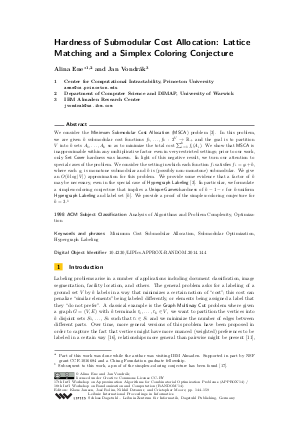Document# Hardness of Submodular Cost Allocation: Lattice Matching and a Simplex Coloring Conjecture

### Authors Alina Ene, Jan Vondrák## File

LIPIcs.APPROX-RANDOM.2014.144.pdf
• Filesize: 0.52 MB
• 16 pages

## Cite As

Alina Ene and Jan Vondrák. Hardness of Submodular Cost Allocation: Lattice Matching and a Simplex Coloring Conjecture. In Approximation, Randomization, and Combinatorial Optimization. Algorithms and Techniques (APPROX/RANDOM 2014). Leibniz International Proceedings in Informatics (LIPIcs), Volume 28, pp. 144-159, Schloss Dagstuhl - Leibniz-Zentrum für Informatik (2014)
https://doi.org/10.4230/LIPIcs.APPROX-RANDOM.2014.144

## Abstract

We consider the Minimum Submodular Cost Allocation (MSCA) problem. In this problem, we are given k submodular cost functions f_1, ... , f_k: 2^V -> R_+ and the goal is to partition V into k sets A_1, ..., A_k so as to minimize the total cost sum_{i = 1}^k f_i(A_i). We show that MSCA is inapproximable within any multiplicative factor even in very restricted settings; prior to our work, only Set Cover hardness was known. In light of this negative result, we turn our attention to special cases of the problem. We consider the setting in which each function f_i satisfies f_i = g_i + h, where each g_i is monotone submodular and h is (possibly non-monotone) submodular. We give an O(k log |V|) approximation for this problem. We provide some evidence that a factor of k may be necessary, even in the special case of HyperLabel. In particular, we formulate a simplex-coloring conjecture that implies a Unique-Games-hardness of (k - 1 - epsilon) for k-uniform HyperLabel and label set [k]. We provide a proof of the simplex-coloring conjecture for k=3.
##### Keywords
• Minimum Cost Submodular Allocation
• Submodular Optimization
• Hypergraph Labeling

## Metrics

• Access Statistics
• Total Accesses (updated on a weekly basis)
0